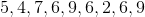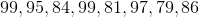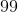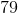ISEE Upper Level Math : How to find mode

Example Questions

1 3 Next →

Example Question #21 : How To Find Mode

Use the following data set to answer the question:Find the mode.Explanation:

To find the mode of a data set, we will find the number that appears most often.

So, given the setwe can see the number that appears most often is 6 (it appears 3 times).

Therefore, the mode of the data set is 6.

Example Question #22 : How To Find Mode

Use the following data set of test scores to answer the question:Find the mode.Explanation:

To find the mode of a data set, we will find the number the appears most often.

So, given the setWe can see the number that appears most often is 99.

Therefore, the mode of the data set is 99.

1 3 Next →